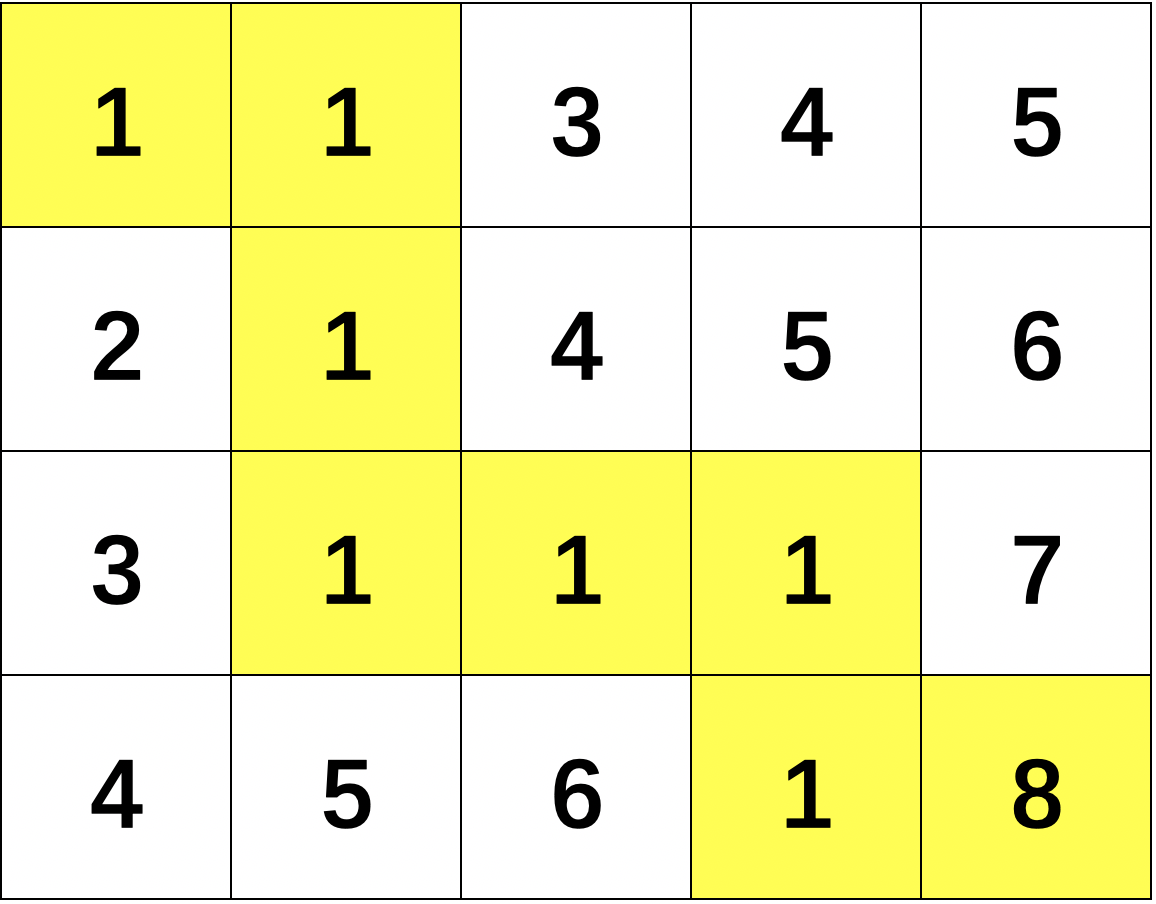# 算法刷题-面试刷题练手

## 题1

def max_seq(nums):
p_first = 0
p_next = p_first
p_max = p_first

sum_current = 0
sum_max = sum_current

for e in nums:
if p_next < p_first:
p_next = p_first

if sum_current + e <= 0:
p_first = p_next + 1
sum_current = 0
else:
sum_current = sum_current + e

p_next = p_next + 1

if sum_current > sum_max:
sum_max = sum_current
p_max = p_next

return (sum_max, p_first, p_max)

if __name__ == '__main__':
nums = [-2, 1, -3, 4, -1, 2, 1, -5, 4]

sum_max, p_first, p_next = max_seq(nums)

print(sum_max, p_first, p_next, nums[p_first:p_next], sep='\t')


## 题2

#!/usr/bin/python3
# coding:utf-8

def convert(inputStr, k):
"""
convert string.
"""
outputStr = ""

n = len(inputStr)

i = 0

while i * k < n:
if i % 2 == 0:
outputStr += inputStr[ i*k : (i+1)*k ][::-1]
else:
outputStr += inputStr[ i*k : (i+1)*k ]

i += 1

return outputStr

if __name__ == '__main__':
inputStr = "abcdefg"

# 当 k = 2 时
print(convert(inputStr, 2))

# 当 k = 8 时
print(convert(inputStr, 8))


## 题3#!/usr/bin/python3
# coding:utf-8

def dp(m, n, matrix):
"""
DP algorithm.
"""

# 递归的出口
if (m == 0) and (n == 0):
return matrix[m][n]

else:
if (m > 0) and (n > 0):
return matrix[m][n] + min( dp(m-1, n, matrix), dp(m, n-1, matrix) )

elif (m > 0) and (n == 0):
return matrix[m][n] + dp(m-1, n, matrix)

elif (m == 0) and (n > 0):
return matrix[m][n] + dp(m, n-1, matrix)

if __name__ == '__main__':
matrix = [ [1, 1, 3, 4, 5],
[2, 1, 4, 5, 6],
[3, 1, 1, 1, 7],
[4, 5, 6, 1, 8] ]

m = len(matrix)     # 行数
n = len(matrix)  # 列数

min_path_value = dp(m-1, n-1, matrix)

print(min_path_value)


## 题4#!/usr/bin/python3
# coding:utf-8

# 回溯法-八皇后问题所有可能

def place(row, column):
"""
如果一个皇后能放在第row行和column[row]列，则返回true；否则返回false。
前row行已放置皇后
"""

i = 0

while i < row:
# 不允许同一列；不允许对角线
if column[i] == column[row] or abs(column[i]-column[row]) == abs(i-row):
return False

i += 1

return True

def n_queen(row, column, n):
"""
n皇后所有解
"""
# 初始化放置第一列
column[row] = 0

while column[row] < n:
if place(row, column):
# 最后一行放置成功
if row == n - 1:
print( column )
else:
# 下一行
n_queen( row + 1, column, n )

# 下一列
column[row] = column[row] + 1

if __name__ == '__main__':
# 棋盘的大小
n = 8

# 棋盘上的行
row = 0

# 初始化，第 row 行，第 column[row] 列放置皇后
column =  * n

n_queen(row, column, n)